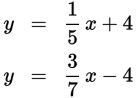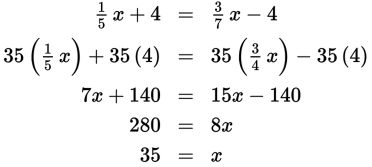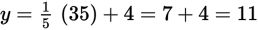# SAT Math Multiple Choice Question 801: Answer and Explanation

### Test Information

Question: 801

6.If the ordered pair (x, y) satisfies the system of equations above, what is the value of y?

• A. 0
• B. 7
• C. 10
• D. 11

Explanation:

D

Difficulty: Medium

Category: Heart of Algebra / Systems of Linear Equations

Strategic Advice: When both equations in a system are written in the form y = mx + b, use substitution or graphing to find the solution to the system. Use whichever method gets you to the answer more quickly.

Getting to the Answer: Substitute the first expression for y into the second equation. Then clear the fractions by multiplying everything by 35 to make the numbers easier to work with, and solve for x:The question asks for the value of y, so substitute 35 into either of the original equations and solve for y:, which is (D).